# Passive Filter Design

A passive ﬁlter component is a combination of capacitors and inductors that are tuned to resonate at a single frequency, or through a band of frequencies. In power systems, passive ﬁlters are used to suppress harmonic currents and decrease voltage distortion appearing in sensitive parts of the system.

Passive ﬁlters work by exhibiting diﬀerent impedance values at the resonant frequency. A ﬁlter connected in series should present high impedance to the harmonic frequency that needs to be blocked. Although a series conﬁguration is possible, it is more common to connect ﬁlters in parallel. Such a shunt conﬁguration diverts harmonic currents to ground, and simultaneously provide reactive power, which may be used to correct the power factor. As such, passive shunt ﬁlters are designed to be capacitive at the fundamental frequency.

## Harmonic Filter Design Procedure

In order to design a harmonic filter, information about the local power system, including environmental data, is required. Power system information includes characteristics, such as the nominal line-to-line voltage, typical equipment BIL for the system voltage level, fundamental frequency, system configuration, and impedance of system components. A clear understanding of equipment location (i.e. indoor or outdoor), operating constraints, equipment current duty-cycle, switching operation rates, environmental data (such as ambient temperature and wind loading), harmonic measurements or manufacturer harmonic characteristics, is important to consider before starting the filter design process.

The IEEE Guide for Application and Specification of Harmonic Filters [??] specifies the following steps as part of the harmonic filter design procedure:

### Determine Harmonic Filter Bank kVAr Size

As described previously, the filter equipment provides the system with capacitive, reactive power for power factor improvement, which helps maintain voltage during heavy loads, in addition to filtering the harmonics. The “Effective kVAr” size of the filter depends on the capacitive, reactive power requirements for power factor, as well as voltage control,  where the required value is commonly determined by power flow programs.

### Select Initial Harmonic Filter Tuning

Filter tuning is necessary to reduce harmonic voltage and current distortion, in order to meet the required harmonic performance. The harmonic filter will typically be tuned to the lower frequency of the most significant harmonic. However, it is recommended that the harmonic filter be tuned between 3 to 15% below the desired frequency to provide sufficient harmonic filtering, and also to allow room for possible filter detuning. Harmonic filter performance across the whole frequency spectrum, at the filter location side (for both normal and contingency conditions) should be considered.

The main reason for tuning the filter below the desired harmonic is due to the nature of filter interaction with the system. That is, the filter itself can shift the parallel resonant frequency very close to the harmonic. This can occur due to a change in filter and system configuration: Natural system variations, such as loss of transformers, equipment replacement or routine maintenance; manufacturer tolerances in both filter reactors and capacitors, capacitor changes due to temperature variations; harmonic filter capacitor unit/element failure due to fuse operation (reduces the total capacitance and increases the filter resonant frequency).

If the level of harmonic distortion is not crucial, the customer may want to avoid overloading the harmonic filter capacitor with harmonic currents, without creating resonance with the system. Then the filter has to be ungrounded to avoid 3rd harmonic resonance with system and tuned below the 5th harmonic to avoid resonance at the characteristic harmonic.

### Optimize the Harmonic Filter Configuration to Meet Harmonic Guidelines

The IEEE Standard 519-1992 provides recommendations to satisfy harmonic distortion limits. The filter is supposed to meet current and voltage distortion requirements for normal system configuration, as well as abnormal conditions. In cases wehere the distortion levels remain high, a new parallel resonance with the system may be a possible cause. Filter re-tuning or multiple-tuned harmonic filter installations can help to solve this problem.

The number of harmonic filters, filter tuning and side locations, values of capacitance, inductance and reactance, allowable tolerances for capacitance, inductance and resistance, Q (X/R ratio) of the harmonic filter reactor at the tuned frequency, as well as steady-state energy dissipation requirements for the harmonic filter resistors, can be the results of the harmonic study analysis.  The harmonic voltage and current spectra, with fundamental and all significant harmonic frequencies for normal and contingency system conditions, is also important outcome of the analysis.

There is a common practice to use a harmonic simulation programs for possible operating conditions over frequency spectrum of the harmonic loads, meanwhile for a simple system this can be manually estimated.

### Determine the Component Ratings

Harmonic filter capacitor rating is usually the first rating to be determined, followed by the reactor, resistor and switch ratings. This process requires adjustments and usually is iterative in nature. Transient simulation studies may also be required for some harmonic filter configurations.

1. Harmonic Filter Capacitors: Harmonic filter capacitors are rated according to voltage and kVAr and are usually given by the manufacturer; depending on the harmonic spectra, transient over-voltages, VAr requirements and system data.  Rated kVAr of the capacitor bank is not equal to effective reactive power because of the effect of the filter reactor.

The capacitor voltage rating is determined from the greatest voltage value at steady-state (including harmonics), transients associated with filter switching and sometimes circuit breaker operation (lasting less than half a cycle) and mechanical dynamics (lasting up to several seconds). In most single-tuned applications, the harmonic filter capacitor voltage is based on steady-state duties. The highest peak voltage applied to the harmonic filter capacitors should not be greater than 100% of peak capacitor voltage rating, for both normal system operation and contingency cases.

For the specified rated kVAr and voltage, the total RMS current through the harmonic filter capacitors should be less than 135% of the capacitor unit normal current, and the current should be kept within the capability of the capacitor fuses. An additional check includes the estimation of dielectric heating of the harmonic filter capacitor. Moreover, it is desirable to have a harmonic filter with more capability than required by harmonic loads and a generous margin is generally included in filter design stage.

1. Harmonic Filter Reactors: The physical location of the harmonic filter reactor, with respect to the harmonic filter capacitor, has to be identified, as it influences thermal issues, magnetic flux heating, reactor short-circuit rating and reactor BIL.

Excessive heating due to fundamental and harmonic currents leads to component degradation , which can be a serious issue for iron-core harmonic filter reactors installed within metal-enclosed filters. The effect of eddy current losses, induced in metallic structures, by an air-core filter reactors magnetic flux has to be analysed at the design stage.

The BIL rating of the phase-to-ground insulation is supposed to be the same as it is for the transformer connected at the same voltage level. Exceptions can be made for filter coils protected by surge arresters, as well as for solidly grounded harmonic filters with the reactor terminal connected to the ground.

Similar to the process of harmonic filter capacitance, levels of transient and dynamic over voltages should be checked. Short-circuit current analysis must be performed for the condition of maximum operating voltage, in order to check the short-current capability for the harmonic filter reactor.

1. Filter Resistors: Physical location of the harmonic filter resistor, with respect to the harmonic filter capacitor must be identified, as it influences thermal issues, resistor short-circuit rating and BIL.

Excessive heating leading to component degradation, due to fundamental and harmonic currents, can be a serious issue for metal-enclosed filters. Animal protection measures are also important considerations for outdoor installations.

Similar to the inductance case, the BIL rating requirements depend on the resistor position within the filter. The short-circuit filter resistor capability should be designed to withstand maximum possible short-circuit current. Magnitudes and durations of transient and dynamic over voltages must be identified and compared to resistor rating. Arrester protection should be included in case of parameter violation.

1. Circuit Breaker or Switch: The capacitive switching requirements should be based on the worst case scenario conditions with maximum system voltage, capacitive tolerances and harmonics. Short-circuit analysis is supposed to include close-and-latch and momentary currents check, and a capacitor switch must handle short-circuit current level, although a capacitor switch, as opposed to circuit breaker, is not supposed to interrupt it.  Moreover, it is important to remember that filter banks develop higher recovery voltage across a switch compared to a shunt capacitor bank. So it is necessary to be aware of choosing the switching device.

1. Switching Transients: It is desirable to provide the designer with data (oscillograms) from transient study analysis, but this is not easy to perform due to the randomness of the events. However, it is possible to model some worst-case harmonic loading scenarios to identify magnitudes and duration of the current by the harmonic order at the worst part of the transient. Some typical scenarios are discussed in the following paragraphs.

Because of the existence of the harmonic filter tuning reactor, back-to-back switching current magnitudes are lower compared to shunt reactor banks and so additional current limiting reactors do not need to be installed. In normal situations, the switching of the single-tuned harmonic filter does not require unusual duty for capacitors or reactors, which are expected for the shunt capacitor bank. Nevertheless, transient performance analysis for initial energization can be required for filters with several legs, to be sure that no high currents or voltages are expected.

If the filter is connected to the system and is supposed to be energized after a system outage, harmonics due to transformer saturation can cause short-time overloading of the harmonic filter. The similar effect on filter can be a result of nearby faults causing transformer saturation and filter overloading. Transient studies to determine harmonic filter performance can be highly desirable.

## Master Library Models

The master library contains four ﬁlter blocks: A series ﬁlter, a high-pass ﬁlter, a band-pass ﬁlter and a c-type ﬁlter. All four filter configurations are illustrated in Figure F-1.

Series filters are also known as single-tuned or notch filters, and it is the type most used in industry. High-pass filters are used to suppress a wider range of frequencies than the single tuned filter, reducing the size of the components and avoiding capacitive power factor when the system is not loaded. Band-pass filters are not common in the industry, but the component can be used to model high-order filters or double-tuned filters.  The c-type filter is a second-order filter, which is designed to have an impedance characteristic similar to the single-tuned filter, with the advantage of having lower power losses.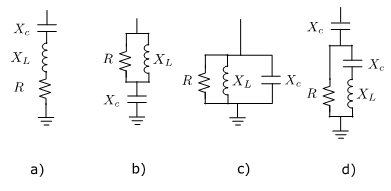Figure F-1:  Filter Configurations. a) Series, b) High-Pass, c) Band-Pass, d) C-Type

## Series (Single-Tuned) Filter

Figure F-2 shows the circuit schematic and a typical impedance characteristic for the series or single-tuned filter.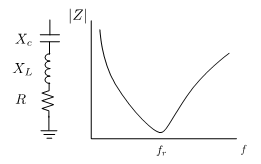Figure F-2:  Series (Single-Tuned) Filter

This ﬁlter is tuned to suppress a single frequency and is designed based on three quantities: The harmonic current order that requires blocking, the capacitive reactive power that it is going to provide, and its quality factor. The voltage level and the fundamental frequency, which are given by the system, must also be considered during the design process. In summary, the values used to deﬁne the input parameters are:

 h Tuning point of the ﬁlter (harmonic order) Qc Reactive power of the ﬁlter [MVAr] Q Quality factor f System frequency [Hz] V System voltage [kV]

The quality factor is a quantity that defines the bandwidth of the ﬁlter and, in this case, is expressed as the ratio between the reactance and the resistance of the ﬁlter. A typical range for Q is between 30 and 60.

The following equations can be applied for designing the ﬁlter: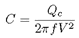(F-1)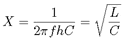(F-2)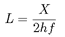(F-3)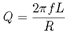(F-4)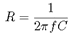(F-5)

Where, X is the reactance of the inductor or the capacitor at the tuned frequency.

### Examples

The following example illustrates the use of the series filter. The circuit in Figure F-3 represents a system that has X/R = 10 (Q) and a load that injects typical harmonic currents generated by a twelve-pulse converter. This type of converter injects high currents for the 11th and 13th harmonics into the AC side. Thus, filters are designed to suppress those currents.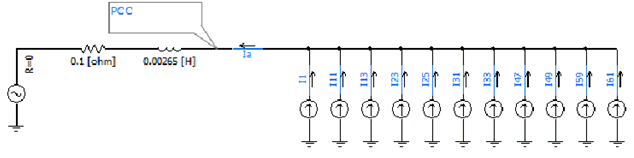Figure F-3:  System Configuration without Filters

In this case, the 11th and 13th harmonic series filters are installed with the parameters described previously.  Figure F-6 illustrates the circuit with the filters installed in the point of common coupling. The Fast Fourier Transform (FFT) is used to measure the current magnitudes for the 11th and 13th harmonic in both circuits.

Using Equations F-1 to F-5, the ﬁlter tuned to the 11th harmonic can be represented by the following quantities: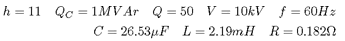The impedance characteristic of this series filter is designed to resonate at the 11th harmonic and is displayed in Figure F-4.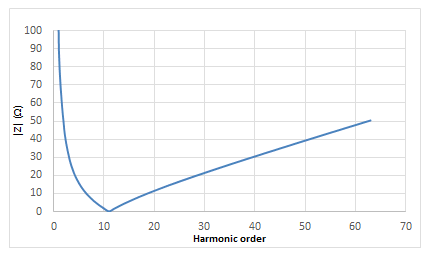Figure F-4: Impedance Characteristics of Series Filters

Figure F-5 shows the impedance characteristic for a combination of 11th and 13th harmonic series filters.  Values for these two filters are:

C11 =13,26 µF , L11 = 4.38 mH, R11 = 0.366 Ω

C13 =13,26 µF, L13 = 3.14 mH, R13 = 0.308 Ω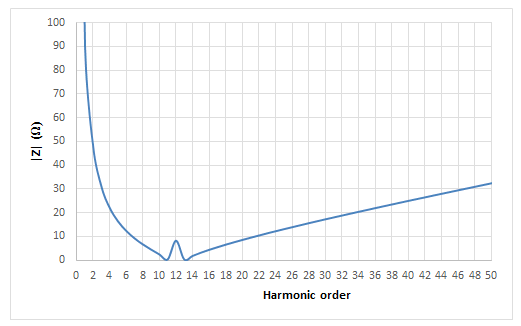Figure F-5: Impedance Characteristic for a Combination of 11th and 13th Harmonic Series Filters

The impedance characteristics are obtained using the Interface to Harmonic Impedance Solution from the master library. The results show a reduction of 96% in the magnitude of the currents.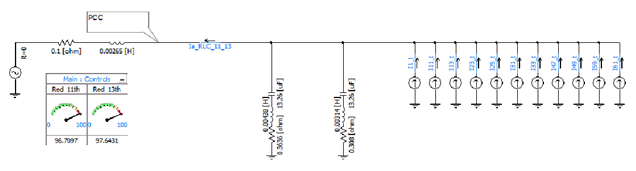Figure F-6:  System Configuration with Series Filters

Figure F-7 shows the improvement in the current waveform injected into the network.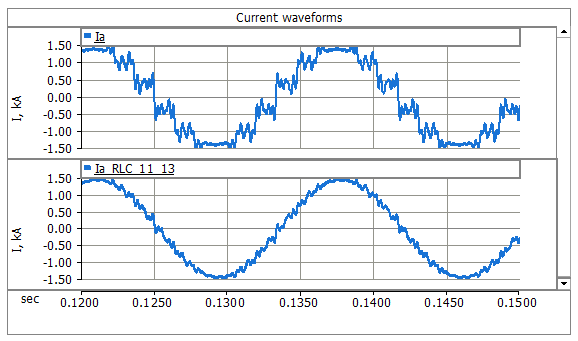Figure F-7:  Current Waveform Comparisons

## High-Pass Filter

Figure F-8 shows the circuit schematic and a typical impedance characteristic for a high-pass filter.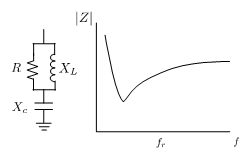Figure F-8:  High-Pass Filter

This filter is designed to have an impedance characteristic that is flat for high frequencies. Looking at the equation that represents the variation of the impedance with the frequency,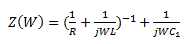(F-6)

It can be shown that low resistance values will increase the losses, due to that parallel connection with the inductor, and having higher inductance is easier to achieve when designing the filter to work at high frequencies. Thus, this type of filter is applied to suppress 5th harmonic order currents or higher.  The resistance also establishes an asymptotic behaviour in the impedance, limiting the maximum value at high frequencies. This means wide bandwidth that can be measured by the quality factor, which is the inverse of that for the series filter, and it is designed to have values between 0.5 and 2.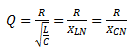(F-7)

Where XLN = XCN are reactances at the tuned frequency.

### Examples

As an example, a high-pass filter tuned for the 11th harmonic, working in a 60 Hz system, was calculated using the Equations F1 to F5.  The reactive power capacity at the fundamental frequency was selected as 1.0 MVAr at 10 kV and a Q of 1. The parameters of the filter are:

C = 26.31 µF   L = 2.21 mH   R = 9.17 W

The frequency response of the filter is depicted in Figure F-9.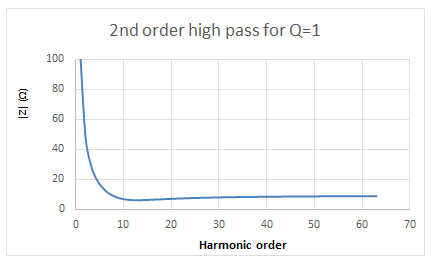Figure F-9:  Impedance Characteristic for High-Pass Filter Tuned to 11th Harmonic

The high-pass filter is designed to work in combination with one, or several notch filters that suppress lower order harmonics, in order to avoid the power losses that the filter creates when it is designed for low frequencies.

## Band-Pass Filter

This component can be used in to model high-order filters. Probably the most popular is the double-tuned filter (depicted in Figure F-10), which is a combination of a band-pass filter in series with an inductor and a capacitor. This type of filter works by combining the parallel resonance of the band-pass filter, with the series-resonance of the inductor and capacitor combination. Two new resonant frequencies are optioned, as shown in the graph. This configuration makes the filter less expensive to build than the parallel combination of two independent series filters.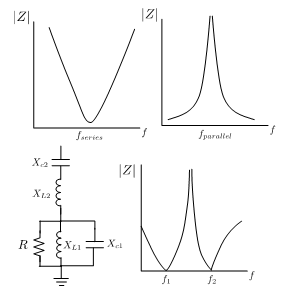Figure F-10:  Impedance Characteristic of a Double-Tuned Filter

## C-Type Filter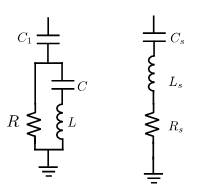Figure F-11:  Equivalent Series Filter for the C-Type Filter

C-type filters are second-order filters that have the capacity of suppressing harmonic currents with lower losses than the series filter or the band-pass filter. The reason for this capability is the  L and C elements, which are parallel with the resistor, resonate at the fundamental frequency. Thus, the fundamental current that circulates through the damping resistor is reduced to a minimum. Another advantage is that c-type filters perform well in suppressing high frequency harmonics, due to their inherently flat impedance characteristic above the tuned frequency.

The design input parameters for the C-type filter are the same as they are for the series filter, and its impedance can be expressed on the basis of  R, L, C, C1: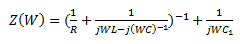(F-8)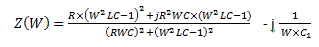(F-9)

According to Reference , the parameters of this filter can be calculated using the model of the series filter, as it is shown in Figure F-11. Using Qc, Q, V and h as inputs, the first step is to calculate the parameters of the series filter elements Ls and Cs. Considering that the inductance L is equal to Ls, the second step is to calculate C to be resonant with L at the fundamental frequency. The capacitor C1 is calculated considering that C and C1 are connected in series and equal to Cs.

The following equations summarized the steps: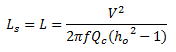(F-10)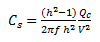(F-11)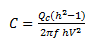(F-12)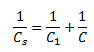(F-13)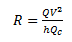(F-14)

### Examples

As for the series filter, the parameters of the C-type filter R, L, C, C1 are calculated based on nominal voltage V, tuning frequency of the filter ωo and the reactive power capacity at fundamental  frequency Qc. Neglecting the resistance in the reactor and the dielectric power loss in the capacitors, the impedance of the filter will be as follows:

Parameters L and C are tuned up to fundamental frequency to avoid power loss at fundamental frequency on damping resistor R: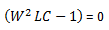(F-15)

The impedance of the filter at fundamental frequency is determined by C1 only: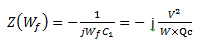(F-16)

Reference  Introduces a practical approach in C-type filter parameters estimation, based on a comparison with a series filter and some simplifications and assumptions. It is suggested that the series filter have the same nominal voltage, reactive compensation power and tuned harmonic as C-type filter.

Series C and L parameters for C-type filter: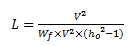(F-17)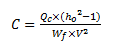(F-18)

The sum of C1 and C of the C-type filter in a series connection is equal to CS of the series filter: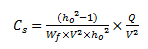(F-19)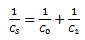(F-20)

The damping resistance using quality factor definition: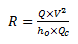(F-21)

For the Quebec-New England Transmission project, the C-type filter, tuned for the 3rd harmonic, was implemented, working in a 60 Hz system with reactive power capacity at fundamental frequency of 49 MVAr at 315 kV and a quality factor of 2.

The C-type filter parameter calculations using Equations F-16 to F-21 give the following results:

R = 1349 W

L = 671 mH

C = 10.48 µF

Cs = 1.164 µF and

C1 = 1.31 µF

The impedance characteristic for the discussed C-type filter is represented in Figure F-12. The graph has been created using the Interface to Harmonic Impedance Solution component from PSCAD master library.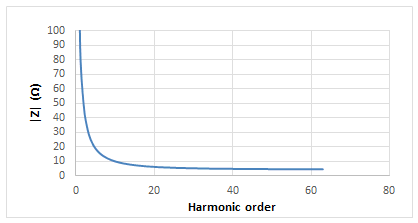Figure F-12:  Impedance Characteristic for a C-Type Filter Tuned to 3rd Harmonic